﻿ MechDesigner Reference & User Interface > 2.2 Mechanism-Editor: > Kinematic Function-Blocks > Kinematic FB | Add Point-Data FB

# Kinematic FB | Add Point-Data FB

### Why use a Point-Data Function-Block?

 The Point FB measures motion-values of a Point or Motion-Point with respect to the Mechanism Plane. It has three output-connectors. From top-to-bottom, they are: ➔X-axis motion-values ➔Y-axis motion-values ➔Magnitude of the motion-values equal to: o√(X2 + Y2) Note: Motion-Values include the Position, Velocity, and Acceleration of the Point or Motion-Point. The Point must be a child to a kinematically-defined Part.

STEP 1: Add the Point-Data FB to the graphic-area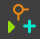1.Click Kinematics FB toolbar > Point-Data FB or 1.Click Add menu > Add Point-Data FB Then: 2.Click the graphic-area

The Point-Data FB is now in the graphic-area (and Assembly-Tree).

Edit :

STEP 2: Open the Point-Data dialog-box: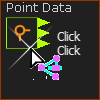1.Double-click the Point-Data FB in the graphic-area - or -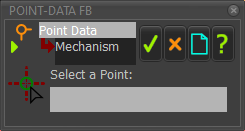Point-DATA FB - with no  Point

STEP 3: Select a Point (or Motion-Point)

 When the box is Select a Point: 1.Click a Point in the graphic-area.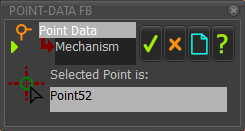Point-Data FB- with a Point

The box is now: Selected Point is:

STEP 4: Close the Point-Data Dialog-box

 1.Click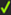in the Point-Data dialog-box

The motion-values for the Point (or Motion-Point) are now at the output-connectors of the Point-Data FB.

How to use the Point-Data FB:

You can connect a wire from the output-connector of a Point-Data FB:

 •To plot the Position, Velocity and Acceleration of the Point with a Graph FB - see Note 1 below •To the input-connector of a Motion FB. The motion-values from the Point-Data FB become the independent variable for a Motion in MotionDesigner, More usually, the independent variable is the output of a Linear-Motion FB - see Note 2 below •To the input-connector of a Motion-Dimension FB - see Note 2 below

Note 1:

To plot the Position, Velocity and Acceleration of the Point in the same graph.

1.Drag a wire from the same output-connector of the Point-Data FB three times to different input-connectors on a Graph FB,

2.Use the Y–axis display options in the Graph Interface to display the three different motion-derivatives.

Note 2:

There will be a message in the Feedback-Area: '1 Mechanism dependencies detected'.

You should rebuild the model when the number of dependencies becomes more than 1 if the data is critical, before you use the data for a Cam, for example.